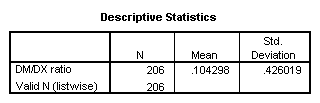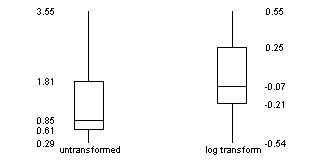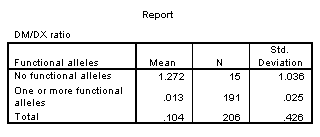# Log transformation

## 2002-10-11

Dear Professor Mean, I have some data that I need help with analysis. One suggestion is that I use a log transformation. Why would I want to do this?

Think of it as employment security for us statisticians.

If you want to use a log transformation, you compute the logarithm of each data value and then analyze the resulting data. You may wish to transform the results back to the original scale of measurement.

The logarithm function tends to squeeze together the larger values in your data set and stretches out the smaller values. This squeezing and stretching can correct one or more of the following problems with your data:

1. Skewed data
2. Outliers
3. Unequal variation

Not all data sets will suffer from these problems. Even if they do, the log transformation is not guaranteed to solve these problems. Nevertheless, the log transformation works surprisingly well in many situations.

Furthermore, a log transformation can sometimes simplify your statistical models. Some statistical models are multiplicative: factors influence your outcome measure through multiplication rather than addition. These multiplicative models are easier to work with after a log transformation.

If you are unsure whether to use a log transformation, here are a few things you should look for:

1. Is your data bounded below by zero?
2. Is your data defined as a ratio?
3. Is the largest value in your data more than three times larger than the smallest value?

Squeezing and stretching

The logarithm function squeezes together big data values (anything larger than 1). The bigger the data value, the more the squeezing. The graph below shows this effect.The first two values are 2.0 and 2.2. Their logarithms, 0.69 and 0.79 are much closer. The second two values, 2.6 and 2.8, are squeezed even more. Their logarithms are 0.96 and 1.03.

The logarithm also stretches small values apart (values less than 1). The smaller the values the more the stretching. This is illustrated below.The values of 0.4 and 0.45 have logarithms (-0.92 and -0.80) that are further apart. The values of 0.20 and 0.25 are stretched even further. Their logarithms are -1.61 and -1.39, respectively.

Skewness

If your data are skewed to the right, a log transformation can sometimes produce a data set that is closer to symmetric. Recall that in a skewed right distribution, the left tail (the smaller values) is tightly packed together and the right tail (the larger values) is widely spread apart.The logarithm will squeeze the right tail of the distribution and stretch the left tail, which produces a greater degree of symmetry.

If the data are symmetric or skewed to the left, a log transformation could actually make things worse. Also, a log transformation is unlikely to be effective if the data has a narrow range (if the largest value is not more than three times bigger than the smallest value).

Outliers

If your data has outliers on the high end, a log transformation can sometimes help. The squeezing of large values might pull that outlier back in closer to the rest of the data. If your data has outliers on the low end, the log transformation might actually make the outlier worse, since it stretches small values.

Unequal variation

Many statistical procedures require that all of your subject groups have comparable variation. If you data has unequal variation, then the some of your tests and confidence intervals may be invalid. A log transformation can help with certain types of unequal variation.

A common pattern of unequal variation is when the groups with the large means also tend to have large standard deviations. Consider housing prices in several different neighborhoods. In one part of town, houses might be cheap, and sell for 60 to 80 thousand dollars. In a different neighborhood, houses might sell for 120 to 180 thousand dollars. And in the snooty part of town, houses might sell for 400 to 600 thousand dollars. Notice that as the neighborhoods got more expensive, the range of prices got wider. This is an example of data where groups with large means tend to have large standard deviations.

With this pattern of variation, the log transformation can equalize the variation. The log transformation will squeeze the groups with the larger standard deviations more than it will squeeze the groups with the smaller standard deviations. The log transformation is especially effective when the size of a group’s standard deviation is directly proportional to the size of its mean.

Multiplicative models

There are two common statistical models, additive and multiplicative.

An additive model assumes that factors that change your outcome measure, change it by addition or subtraction. An example of an additive model would when we increase the number of mail order catalogs sent out by 1,000, and that adds an extra 5,000 in sales.

A multiplicative model assumes that factors that change your outcome measure, change it by multiplication or division. An example of a multiplicative model woud be when an inch of rain takes half of the pollen out of the air.

In an additive model, the changes that we see are the same size, regardless of whether we are on the high end or the low end of the scale. Extra catalogs add the same amount to our sales regardless of whether our sales are big or small. In a multiplicative model, the changes we see are bigger at the high end of the scale than at the low end. An inch of rain takes a lot of pollen out on a high pollen day but proportionately less pollen out on a low pollen day.

If you remember your high school algebra, you’ll recall that the logarithm of a product is equal to the sum of the logarithms.Therefore, a logarithm converts multiplication/division into addition/subtraction. Another way to think about this in a multiplicative model, large values imply large changes and small values imply small changes. The stretching and squeezing of the logarithm levels out the changes.

When should you consider a log transformation?

There are several situations where a log transformation should be given special consideration.

Is your data bounded below by zero? When your data are bounded below by zero, you often have problems with skewness. The bound of zero prevents outliers on the low end, and constrains the left tail of the distribution to be tightly packed. Also groups with means close to zero are more constrained (hence less variable) than groups with means far away from zero.

It does matter how close you are to zero. If your mean is within a standard deviation or two of zero, then expect some skewness. After all the bell shaped curve which speads out about three standard deviations on either side would crash into zero and cause a traffic jam in the left tail.

Is your data defined as a ratio? Ratios tend to be skewed by their very nature. They also tend to have models that are multiplicative.

Is the largest value in your data more than three times larger than the smallest value? The relative stretching and squeezing of the logarithm only has an impact if your data has a wide range. If the maximum of your data is not at least three times as big as your minimum, then the logarithm can’t squeeze and stretch your data enough to have any useful impact.

Example

The DM/DX ratio is a measure of how rapidly the body metabolizes certain types of medication. A patient is given a dose of dextrometorphan (DM), a common cough medication. The patients urine is collected for four hours, and the concentrations of DM and DX (a metabolite of dextrometorphan) are measured. The ratio of DM concentration to DX is a measure of how well the CYD 2D6 metabolic pathway functions. A ratio less than 0.3 indicates normal metabolism; larger ratios indicate slow metabolism.

Genetics can influence CYP 2D6 metabolism. In this set of 206 patients, we have 15 with no functional alleles and 191 with one or more functional alleles.

The DM/DX ratio is a good candidate for a log transformation since it is bounded below by zero. It is also obviously a ratio. The standard deviation for this data (0.4) is much larger than the mean (0.1).Finally, the largest value is several orders of magnitude bigger than the smallest value.Skewness

The boxplots below show the original (untransformed) data for the 15 patients with no functional alleles. The graph also shows the log transformed data. Notice that the untransformed data shows quite a bit of skewness. The lower whisker and the lower half of the box are much packed tightly, while the upper whisker and the upper half of the box are spread widely.

The log transformed data, while not perfectly symmetric, does tend to have a better balance between the lower half and the upper half of the distribution.Outliers

The graph below shows the untransformed and log transformed data for the subset of patients with exactly two functional alleles (n=119). The original data has two outliers which are almost 7 standard deviations above the mean. The log transformed data are not perfect, and perhaps there is now an outlier on the low end. Nevertheless, the worst outlier is still within 4 standard deviations of the mean. The influence of outliers is much less extreme with the log transformed data.Unequal variation

When we compute standard deviations for the patients with no functional alleles and the patients with one or more functional alleles, we see that the former group has a much larger standard deviation. This is not too surprising. The patients with no functional alleles are further from the lower bound and thus have much more room to vary.After a log transformation, the standard deviations are much closer.Summary

Stumped Susan wants to understand why she should use a log transformation for her data. Professor Mean explains that a log transformation is often useful for correcting problems with skewed data, outliers, and unequal variation. This works because the log function squeezes the large values of your data together and stretches the small values apart. The log transformation is also useful when you believe that factors have a mutliplicative effect. You should consider a log transformation when your data are bound below by zero, when you data are defined as a ratio, and/or when the largest value in your data is at least three times as big as the smallest value.